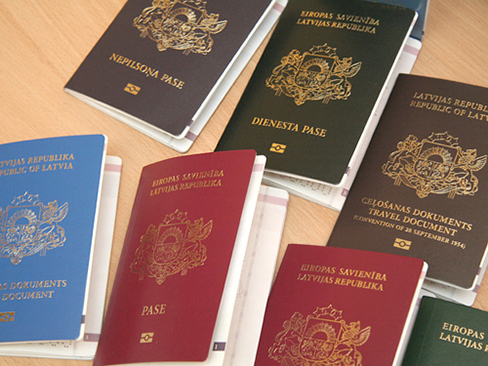# 9.1 Solving trigonometric equations with identities

 Page 1 / 9
In this section, you will:
• Verify the fundamental trigonometric identities.
• Simplify trigonometric expressions using algebra and the identities.International passports and travel documents

In espionage movies, we see international spies with multiple passports, each claiming a different identity. However, we know that each of those passports represents the same person. The trigonometric identities act in a similar manner to multiple passports—there are many ways to represent the same trigonometric expression. Just as a spy will choose an Italian passport when traveling to Italy, we choose the identity that applies to the given scenario when solving a trigonometric equation.

In this section, we will begin an examination of the fundamental trigonometric identities, including how we can verify them and how we can use them to simplify trigonometric expressions.

## Verifying the fundamental trigonometric identities

Identities enable us to simplify complicated expressions. They are the basic tools of trigonometry used in solving trigonometric equations, just as factoring, finding common denominators, and using special formulas are the basic tools of solving algebraic equations. In fact, we use algebraic techniques constantly to simplify trigonometric expressions. Basic properties and formulas of algebra, such as the difference of squares formula and the perfect squares formula, will simplify the work involved with trigonometric expressions and equations. We already know that all of the trigonometric functions are related because they all are defined in terms of the unit circle. Consequently, any trigonometric identity can be written in many ways.

To verify the trigonometric identities, we usually start with the more complicated side of the equation and essentially rewrite the expression until it has been transformed into the same expression as the other side of the equation. Sometimes we have to factor expressions, expand expressions, find common denominators, or use other algebraic strategies to obtain the desired result. In this first section, we will work with the fundamental identities: the Pythagorean identities    , the even-odd identities, the reciprocal identities, and the quotient identities.

We will begin with the Pythagorean identities (see [link] ), which are equations involving trigonometric functions based on the properties of a right triangle. We have already seen and used the first of these identifies, but now we will also use additional identities.

Pythagorean Identities
${\mathrm{sin}}^{2}\theta +{\mathrm{cos}}^{2}\theta =1$ $1+{\mathrm{cot}}^{2}\theta ={\mathrm{csc}}^{2}\theta$ $1+{\mathrm{tan}}^{2}\theta ={\mathrm{sec}}^{2}\theta$

The second and third identities can be obtained by manipulating the first. The identity $\text{\hspace{0.17em}}1+{\mathrm{cot}}^{2}\theta ={\mathrm{csc}}^{2}\theta \text{\hspace{0.17em}}$ is found by rewriting the left side of the equation in terms of sine and cosine.

Prove: $\text{\hspace{0.17em}}1+{\mathrm{cot}}^{2}\theta ={\mathrm{csc}}^{2}\theta$

Similarly, $\text{\hspace{0.17em}}1+{\mathrm{tan}}^{2}\theta ={\mathrm{sec}}^{2}\theta \text{\hspace{0.17em}}$ can be obtained by rewriting the left side of this identity in terms of sine and cosine. This gives

#### Questions & Answers

A laser rangefinder is locked on a comet approaching Earth. The distance g(x), in kilometers, of the comet after x days, for x in the interval 0 to 30 days, is given by g(x)=250,000csc(π30x). Graph g(x) on the interval [0, 35]. Evaluate g(5)  and interpret the information. What is the minimum distance between the comet and Earth? When does this occur? To which constant in the equation does this correspond? Find and discuss the meaning of any vertical asymptotes.
Kaitlyn Reply
The sequence is {1,-1,1-1.....} has
amit Reply
circular region of radious
Kainat Reply
how can we solve this problem
Joel Reply
Sin(A+B) = sinBcosA+cosBsinA
Eseka Reply
Prove it
Eseka
Please prove it
Eseka
hi
Joel
June needs 45 gallons of punch. 2 different coolers. Bigger cooler is 5 times as large as smaller cooler. How many gallons in each cooler?
Arleathia Reply
7.5 and 37.5
Nando
find the sum of 28th term of the AP 3+10+17+---------
Prince Reply
I think you should say "28 terms" instead of "28th term"
Vedant
the 28th term is 175
Nando
192
Kenneth
if sequence sn is a such that sn>0 for all n and lim sn=0than prove that lim (s1 s2............ sn) ke hole power n =n
SANDESH Reply
write down the polynomial function with root 1/3,2,-3 with solution
Gift Reply
if A and B are subspaces of V prove that (A+B)/B=A/(A-B)
Pream Reply
write down the value of each of the following in surd form a)cos(-65°) b)sin(-180°)c)tan(225°)d)tan(135°)
Oroke Reply
Prove that (sinA/1-cosA - 1-cosA/sinA) (cosA/1-sinA - 1-sinA/cosA) = 4
kiruba Reply
what is the answer to dividing negative index
Morosi Reply
In a triangle ABC prove that. (b+c)cosA+(c+a)cosB+(a+b)cisC=a+b+c.
Shivam Reply
give me the waec 2019 questions
Aaron Reply

### Read also:

#### Get the best Algebra and trigonometry course in your pocket!

Source:  OpenStax, Algebra and trigonometry. OpenStax CNX. Nov 14, 2016 Download for free at https://legacy.cnx.org/content/col11758/1.6
Google Play and the Google Play logo are trademarks of Google Inc.

Notification Switch

Would you like to follow the 'Algebra and trigonometry' conversation and receive update notifications?ByByBy